# How to create a numbering AA, AB, AC... instead of AA, BB, CC... in Word

Word
Unfortunately, Word doesn't propose the option to change the pre-defined numeration AA, BB, CC... to the AA, AB, AC... as it required for different cases. This tip proposes step by step guide how to create the necessary numeration AA, AB, AC, etc.: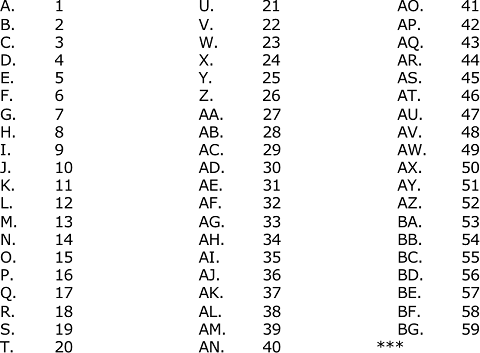Attention! Be very careful, working with Word fields is not simple and require adding formulas step-by-step and avoiding copy-pasting.

To create a numeration AA, AB, AC, etc., you can add the following formula in every line that should be numerated (after typing this formula by the following steps, you can copy and paste it):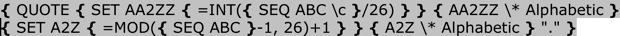Note: You can customize how to show fields in the document, see How to turn on or turn off highlighting of fields in a Word document.

To create a numeration AA, AB, AC, etc., follow the next steps:

1.   Create a field to show the numeration from A to Z repeatedly:

This displays the special brackets with a gray highlight for entering a field name: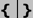1.2.   Type QUOTE in the brackets and then again click Ctrl+F9 to add new field: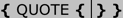The QUOTE field shows the specific text in the document. So, it should be shown the results of the following after QUOTE formulas.

1.3.   In the second field type SET A2Z (A2Z is the name of a variable that will be used to show the last letter of the numeration list):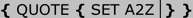The SET field assigns specific information to a named variable. So, the variable with name A2Z (you can use your own name) will be assigned with the result of the following formula.

1.4.   Type again Ctrl+F9 to add he formula to calculate the next value of the numeration list:

= MOD (<argument 1>, <argument 2>) + 1,

The MOD formula takes two arguments (must be numbers or evaluate to numbers). Returns the remainder after the second argument is divided by the first.

For the English alphabetic numeration, use the following formula: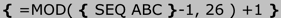The SEQ (Sequence) field sequentially numbers chapters, tables, figures, and other items in a document. In this example we specify the item as ABC – English capital letters by alphabetic.

Sure, the SEQ ABC should be pasted as a field (click Ctrl+F9 to insert the specific brackets and type SEQ ABC inside them).

1.5.   Place the cursor to the end of the field QOUTE to add the second argument in it: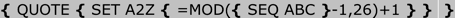1.6.   Click Ctrl+F9 to add new field and type A2Z \* Alphabetic: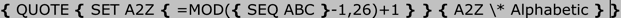Now, if you copy this field, select all copies and update them, you will see the repeated numbering from A to Z: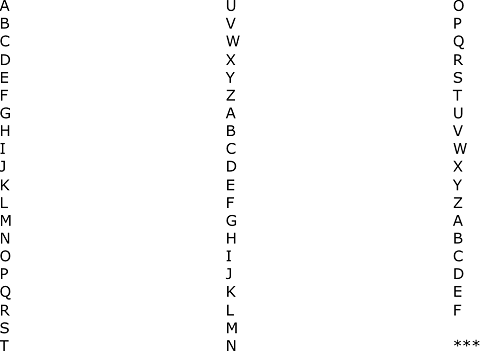Note: To update fields, select them, right-click on the field and choose Update field in the popup menu: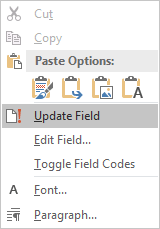2.   Add a first symbol for the numeration AA, AB, AC, etc.:

2.1.   In the QOUTE field, before the inserted fields, click Ctrl+F9 and type SET AA2ZZ (AA2ZZ is the name of the second variable that will be changed to show the first letter of the numeration list).

2.2.   To calculate the letter for the variable AA2ZZ, use the formula:

= INT (<argument>)

The INT formula rounds the value inside the parentheses down to the nearest integer.

For the English alphabetic numeration, use the following formula: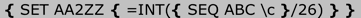The parameter \c in the SEQ field repeats the closest preceding sequence number.

2.3.   To show the variable AA2ZZ, add same fields as added for A2Z: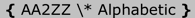Note: To add the point in the end of the numeration, add it in the end of the QUOTE field.

See also this tip in French: Comment créer une numérotation AA, AB, AC ... au lieu de AA, BB, CC ... dans Word.